# Adjoint semi-group of operators

(diff) ← Older revision | Latest revision (diff) | Newer revision → (diff)
Jump to: navigation, search

The semi-group on a dual Banach spacecomposed of the adjoint operators of a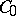-semi-group on(cf. also Semi-group of operators).

Letbe a-semi-group on a Banach space, i.e.for alland

i), the identity operator on;

ii)for all;

iii) the orbits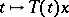are strongly continuous (cf. Strongly-continuous semi-group) onfor all. On the dual space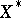, the adjoint semi-group, with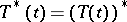, satisfies i) and ii), but not necessarily iii). Therefore one defines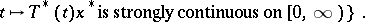This is a norm-closed, weak-dense,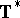-invariant subspace of, and the restrictionis a-semi-group on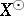, called the strongly continuous adjoint of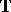. Its infinitesimal generatoris the part of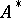in, whereis the adjoint of the infinitesimal generatorof. Its spectrum satisfies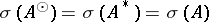. Ifis reflexive (cf. Reflexive space), then[a9].

Starting from, one definesand. The natural mapping,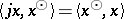, is an isomorphic imbedding with values in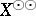, andis said to be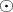-reflexive with respect toifmapsonto. This is the case if and only if the resolventis weakly compact for some (hence for all)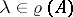[a7]. Ifis-reflexive with respect toand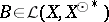, then the part of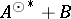ingenerates a-semi-group on[a1].

Let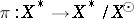be the quotient mapping. If, for some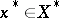, the mappingis separably-valued, thenfor all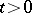. Hence, ifextends to a-group, then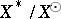is either trivial or non-separable [a4].

Ifis a positive-semi-group on a Banach lattice, thenneed not be a sublattice of[a2]. If, however,has order-continuous norm, thenis even a projection band in[a8]. For a positive-semi-groupon an arbitrary Banach latticeone has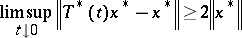for all, the disjoint complement ofin. Ifhas a weak order unit, then for allandone has, the band generated byin[a5]. If, for some, the mapping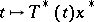is weakly measurable, then, assuming the Martin axiom (cf. Suslin hypothesis), for allone has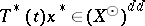[a6].

A general reference is [a3].